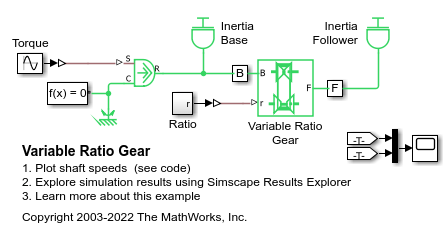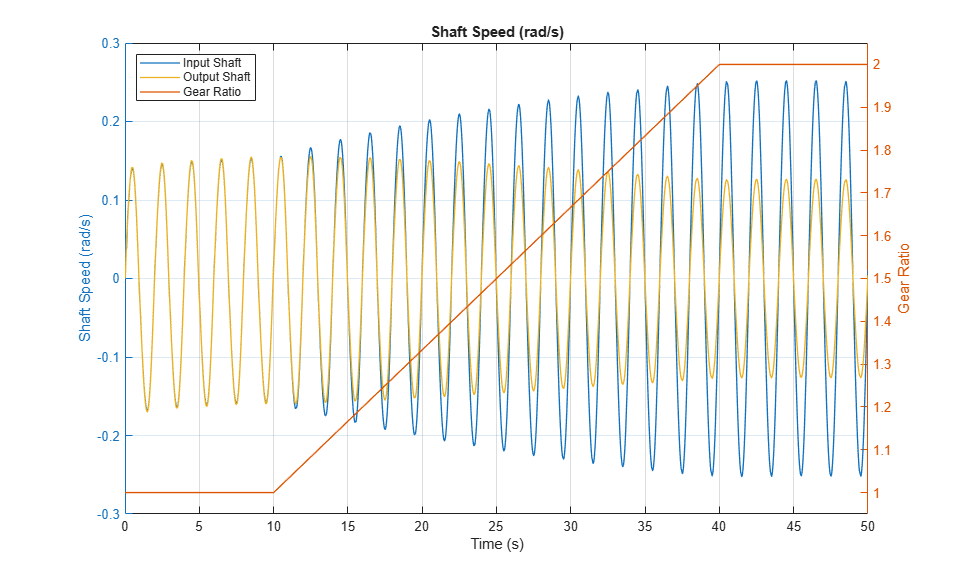# Variable Ratio Gear

This example shows a variable ratio gear coupling two inertias (shafts). The gear ratio between the follower (F) and the base (B) is steadily increased from 1:1 to 2:1. The ratio of the follower to base angular speed decreases with gear ratio, and conversely the ratio of the follower to base torque increases with gear ratio. The variable gear is implemented using the Variable Ratio Transmission block which includes compliance. Appropriate stiffness and damping values for the compliance will depend upon the levels of torque transmitted by the variable gear. If the compliance damping is unrealistically low, the system can develop high frequency oscillations that require small simulation time steps.

### Model### Simulation Results from Simscape Logging

The plot below shows the input and output shaft speeds for a variable ratio gear that is driven by an oscillating torque source. As the ratio increases, the effective inertia decreases and the input shaft maximum speed increases.